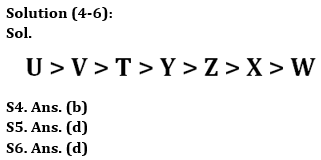Latest Banking jobs   »

# Reasoning Ability Quiz For Bank Foundation 2023 -12th May

Directions (1-3): Answer the questions based on the information given below:
Six persons Amanat, Badshah, Chirag, Damodar, Elvi & Farooq have different weights. Badshah is heavier than two persons. Elvi is lighter than Chirag but not than Farooq. Neither Farooq nor Amanat is the lightest. Amanat is not heavier than Elvi.

Q1. Who among the following is 2nd lightest?
(a) Amanat
(b) Farooq
(c) Damodar
(d) Elvi
(e) Either Farooq or Amanat

Q2. How many persons are lighter than Farooq?
(a) One
(b) Five
(C) Three
(d) Four
(e) Can’t be determined

Q3. Who among the following is 2nd heaviest?
(a) Elvi
(b) Amanat
(c) Farooq
(d) Chirag
(e) Damodar

Directions (4-6): Answer the questions based on the information given below.
There are seven persons T, U, V, W, X, Y & Z with different heights. V is shorter than only one person. Height of Z is the average of heights of V & W. T is taller than Y who is not shorter than Z. Z is taller than X. U is not shorter than T. X is not the shortest.

Q4. What will be the height of the shortest person, if the height of 2nd tallest person is 250cm & Height of Z is 210cm?
(a) 160cm
(b) 170cm
(c) 180cm
(d) 190cm
(e) 200cm

Q5. How many persons are taller than Y?
(a) Two
(b) Either two or three
(c) Four
(d) Three
(e) None of the above

Q6. How many persons are smaller than T?
(a) Five
(b) Two
(c) Three
(d) Four
(e) None of the above

Direction (7-10): In the following questions assuming the given statement to be true, find which of the conclusion(s) among given conclusions is/are definitely true and then give your answers accordingly.

Q7. Statements: F ≤ S ≤ H = J, K = I > C ≥ R, J < K
Conclusions: I. F ≤ K II. S < I
(a) Only I is true
(b) Only II is true
(c) Either I or II is true
(d) Neither I nor II is true
(e) Both I and II are true

Q8. Statements: I = N < O, T > F ≤ I, K = H ≥ T
Conclusions: I. K > F II. F < O
(a) Only I is true
(b) Only II is true
(c) Either I or II is true
(d) Neither I nor II is true
(e) Both I and II are true

Q9. Statements: B = S > E, R = D < K ≥ B, G > T ≥ R
Conclusions: I. K ≥ S II. T ≤ B
(a) Only I is true
(b) Only II is true
(c) Either I or II is true
(d) Neither I nor II is true
(e) Both I and II are true

Q10. Statements: N = C, E ≥ G = H, C > E, A < K ≤ N
Conclusions: I. K ≤ E II. N > H
(a) Only I is true
(b) Only II is true
(c) Either I or II is true
(d) Neither I nor II is true
(e) Both I and II are true

Solutions

Solution (1-3):
Sol. Chirag > Elvi > Amanat/Farooq > Badshah > Farooq/Amanat > Damodar

S1. Ans. (e)
S2. Ans. (e)
S3. Ans. (a)Solution (7-10):
S7. Ans. (b)
Sol. I. F ≤ K – False
II. S < I – True

S8. Ans. (e)
Sol. I. K > F – True
II. F < O – True

S9. Ans. (a)
Sol. I. K ≥ S – True
II. T ≤ B – False

S10. Ans. (b)
Sol. I. K ≤ E – False
II. N > H – True## FAQs

### What is the selection process of the Bank Clerk?

The selection process of the Bank Clerk is Prelims & Mains.

#### Congratulations!Union Budget 2023-24: Free PDF